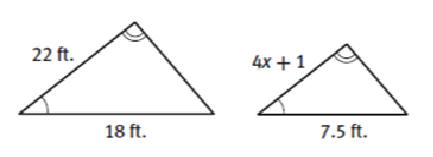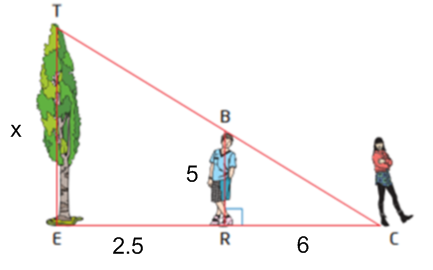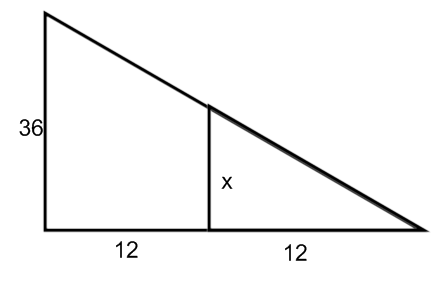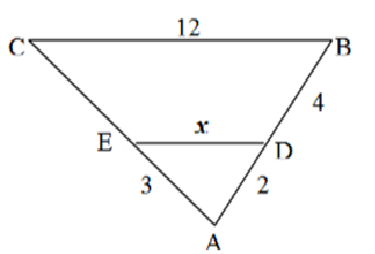GeoB - ACT like similarity
period *
The smaller triangle has sides of 3,4,5. The scale factor between this triangle and a bigger one is 3:1. What is the perimeter of the bigger triangle? *
1 point
set up a proportion to solve for x. *
1 pointA 5ft boy casts a shadow of 6ft. Find the shadow of a 24ft building. *
1 point
last name only *
Use the similarity statement to find x. setup proportions *
1 pointFind shadow of the tree rounded to nearest foot *
1 pointfind x *
1 pointfind x *
1 point